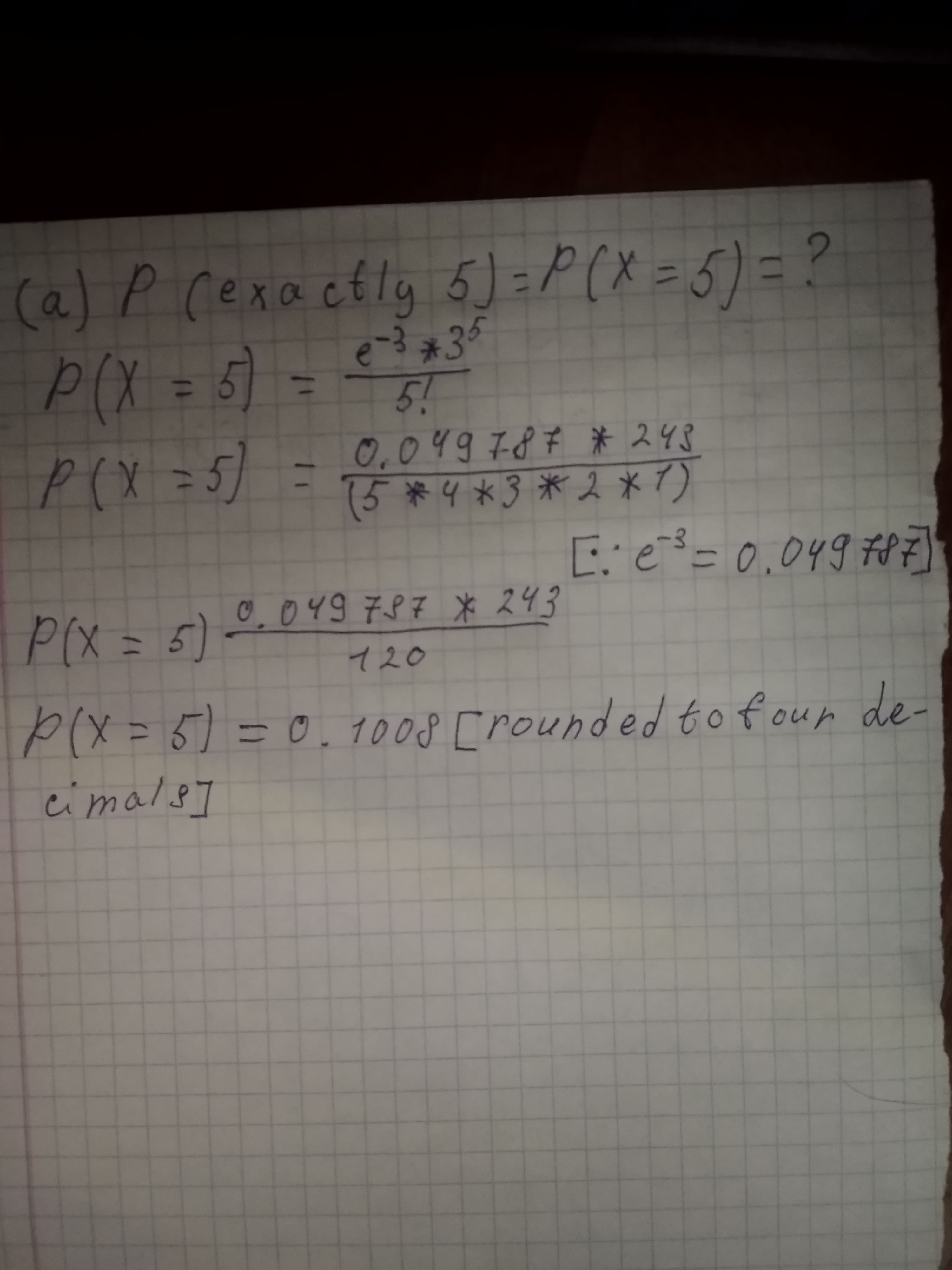# On average, 3 traffic accidents per month occur at a certain intersection. What is the probability that in any given month at this intersection (a) exactly 5 accidents will occur? (b) fewer than 3 accidents will occur? (c) at least 2 accidents will occur?Ayaana Buck 2020-10-21 Answered
On average, 3 traffic accidents per month occur at a certain intersection. What is the probability that in any given month at this intersection
(a) exactly 5 accidents will occur?
(b) fewer than 3 accidents will occur?
(c) at least 2 accidents will occur?
You can still ask an expert for help

• Questions are typically answered in as fast as 30 minutes

Solve your problem for the price of one coffee

• Math expert for every subject
• Pay only if we can solve itberggansS

Given: mean $\left(\lambda \right)=3$
let "X" be the accidents per month occur at a certain intersection
$X-Poisson\left(\lambda =3\right)$
formula:
a)b)c)###### Not exactly what you’re looking for?Jeffrey Jordon

We will assume that the number of accidents at the intersection follows a Poisson distribution with a mean (\mu) of 3 per month

$a\right)P\left[X=5\right]=p\left(5\right)=\frac{{e}^{-\mu }{\mu }^{x}}{x!}=\frac{{e}^{-3}{3}^{5}}{5!}=0.10082\approx 0.10$

$b\right)P\left[X<3\right]=F\left(2\right)=\sum _{x=0}^{2}\frac{{e}^{-\mu }{\mu }^{x}}{x!}=\sum _{x=0}^{2}\frac{{e}^{-3}{3}^{x}}{x!}=0.4232$

$c\right)P\left[X\ge 2\right]=1-P\left[X<2\right]=1-P\left[X\le 1\right]=1-F\left[1\right]=1-\left(\frac{{e}^{-3}{3}^{0}}{0!}+\frac{{e}^{-3}{3}^{1}}{1!}\right)=0.800852\approx 0.8$

###### Not exactly what you’re looking for?

a)

λ=3, t=1, e=2.71828, X=5

=(e^(-3))*(3^(5)) ÷ 5!

=12.0982576134 ÷ 120

=0.10081881344

b)

X=0,1,2 as X<3

p(0;3)+p(1;3)+p(2;3)

p(0;3) = (e^(-3))*(3^(0)) ÷ 0! = 0.04978706836 / 1 = 0.04978706836

p(1;3) = (e^(-3))*(3^(1)) ÷ 1! = 0.1493612051

p(2;3) = (e^(-3))*(3^(2)) ÷ 2! = 0.44808361531 / 2 = 0.22404180765

p(0;3) + p(1;3) + p(2;3) = 0.04978706836 + 0.1493612051 + 0.22404180765 = 0.42319008111

c)

X>=2

[1- ( p(0;3) + p(1;3) + p(2;3) ) ]

As I already derived the values for p(0;3), p(1;3) & p(2;3)

I can directly solve it :

1 - 0.42319008111 = 0.57680991889